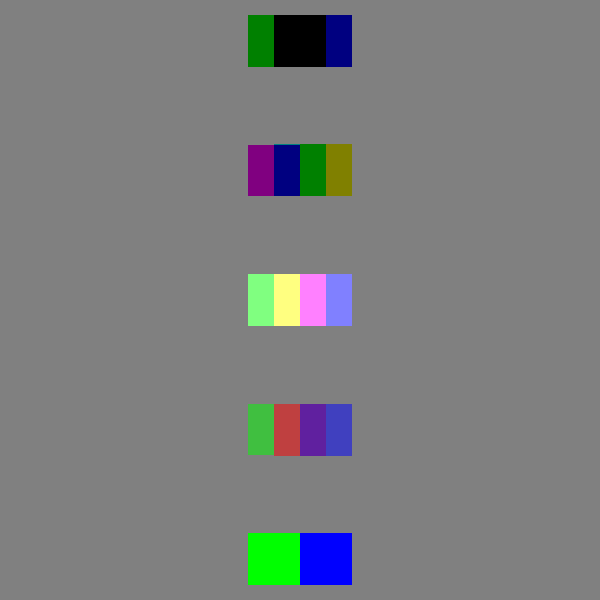# Fine-tuning the OpenGL state using shader callbacks¶

Sometimes we need to get more control about how OpenGL will render the actors. This example shows how to change the OpenGL state of one or more actors. This can be useful when we need to create specialized visualization effects.

First, let’s import some functions

```import numpy as np

from fury.utils import remove_observer_from_actor
from fury import window, actor
import itertools
```

We just proceed as usual: creating the actors and initializing a scene in FURY

```centers = np.array([
[0, 0, 0],
[-.1, 0, 0],
[.1, 0, 0]
])
colors = np.array([
[1, 0, 0],
[0, 1, 0],
[0, 0, 1]
])

actor_no_depth_test = actor.markers(
centers,
marker='s',
colors=colors,
marker_opacity=.5,
scales=.2,
)
actor_normal_blending = actor.markers(
centers - np.array([[0, -.5, 0]]),
marker='s',
colors=colors,
marker_opacity=.5,
scales=.2,
)
centers - np.array([[0, -1, 0]]),
marker='s',
colors=colors,
marker_opacity=.5,
scales=.2,
)

actor_sub_blending = actor.markers(
centers - np.array([[0, -1.5, 0]]),
marker='s',
colors=colors,
marker_opacity=.5,
scales=.2,
)
actor_mul_blending = actor.markers(
centers - np.array([[0, -2, 0]]),
marker='s',
colors=colors,
marker_opacity=.5,
scales=.2,
)

scene = window.Scene()

scene.background((.5, .5, .5))
showm = window.ShowManager(scene,
size=(900, 768), reset_camera=False,
order_transparent=False)
```

All actors must be added in the scene

```scene.add(actor_no_depth_test)
```

Now, we will enter in the topic of this example. First, we need to create (or use one of the pre-built gl_function of FURY) to change the OpenGL state of a given fury window instance (showm.window).

Here we’re using the pre-build FURY window functions which has already a set of specific behaviors to be applied in the OpenGL context

```shader_apply_effects(
showm.window, actor_normal_blending,
effects=window.gl_set_normal_blending)

# ###############################################################################
#  It's also possible use a list of effects. The final opengl state it'll
#  be the composition of each effect that each function has in the opengl state

showm.window, actor_no_depth_test,
effects=[
window.gl_reset_blend, window.gl_disable_blend,
window.gl_disable_depth])

effects=[
window.gl_reset_blend,

showm.window, actor_sub_blending,
effects=window.gl_set_subtractive_blending)

showm.window, actor_mul_blending,
effects=window.gl_set_multiplicative_blending)
```

Out:

```5
```

Finaly, just render and see the results

```showm.initialize()
counter = itertools.count()

# After some steps we will remove the no_depth_test effect

def timer_callback(obj, event):
cnt = next(counter)
showm.render()
# we will rotate the visualization just to help you to see
# the results of each specifc opengl-state
showm.scene.azimuth(1)
if cnt == 400:
remove_observer_from_actor(actor_no_depth_test, id_observer)
showm.window, actor_no_depth_test,
if cnt == 1000:
showm.exit()

interactive = False